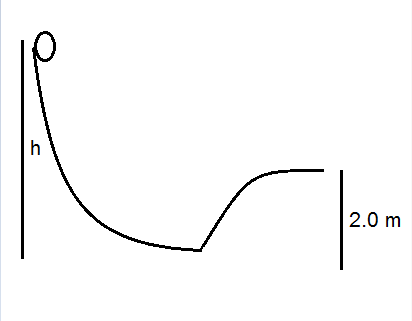A solid sphere starts from rest at the top of a hill of height h. It rolls without slipping down...

Question:

A solid sphere starts from rest at the top of a hill of height {eq}h{/eq}. It rolls without slipping down the hill and then up a smaller hill of height {eq}2.0 m.{/eq} The radius of curvature of the small hill is {eq}9.0 m. {/eq}
a) What is the maximum initial height {eq}h {/eq} such that the ball does not leave the ground at the top of the small hall?
b) What would your answer be if the sphere was hollow?Conservation Of energy:

There are different forms of energy like potential, energy, kinetic energy, rotational energy, etc and according to the conservation of energy these forms of energy are interchangeable for example when a person is standing on the hill then it possesses potential energy and when it is allowed to fall down the hill then the potential energy inside the person changes into kinetic energy and if the object is circular in shape then the potential energy is converted into translational and rotational kinetic energy.

From the conservation of energy potential energy at point A will be equal to the translational kinetic energy and rotational kinetic energy of the ball:

{eq}mg(h-2)=\frac{1}{2}mv^2+\frac{1}{2}I\omega^2..........(1) {/eq}

Here the velocity at point C can be given as:

{eq}v=\sqrt{rg} {/eq}

Here, the radius of curvature of the small hill is {eq}r {/eq}

Acceleration due to gravity is {eq}g {/eq}

Solving for the velocity:

{eq}v=\sqrt{rg}\\ v=\sqrt{9\times 9.81}\\ v=9.39\ m/s {/eq}

Now form the equation (1):

{eq}mg(h-2)=\frac{1}{2}mv^2+\frac{1}{2}I\omega^2\\ mg(h-2)=\frac{1}{2}mv^2+\frac{1}{2}\times \frac{2}{5}mr^2\times \frac{v^2}{r^2}\\ mg(h-2)=\frac{1}{2}\times \frac{7}{5}mv^2\\ mg(h-2)=\frac{1}{2}\times \frac{7}{5}m\times (9.39)^2\\ 9.81\times (h-2)=61.72\\ h-2=6.29\\ h=8.29\ m {/eq}

Thus, the height of the ball at which the ball does not leave the ground is 8.29 m

(b)

Now if the sphere was hollow:

{eq}I=\frac{2}{3}mr^2 {/eq}

Now from the equation (1):

{eq}mg(h-2)=\frac{1}{2}mv^2+\frac{1}{2}\times \frac{2}{3}mr^2\times \frac{v^2}{r^2}\\ mg(h-2)=\frac{1}{2}\times \frac{5}{3}mv^2\\ mg(h-2)=\frac{1}{2}\times \frac{5}{3}m\times (9.39)^2\\ 9.81\times (h-2)=73.47\\ h-2=7.48\\ h=9.48\ m {/eq}

Thus, the height of the hollow ball at which the ball does not leave the ground is 9.48 m